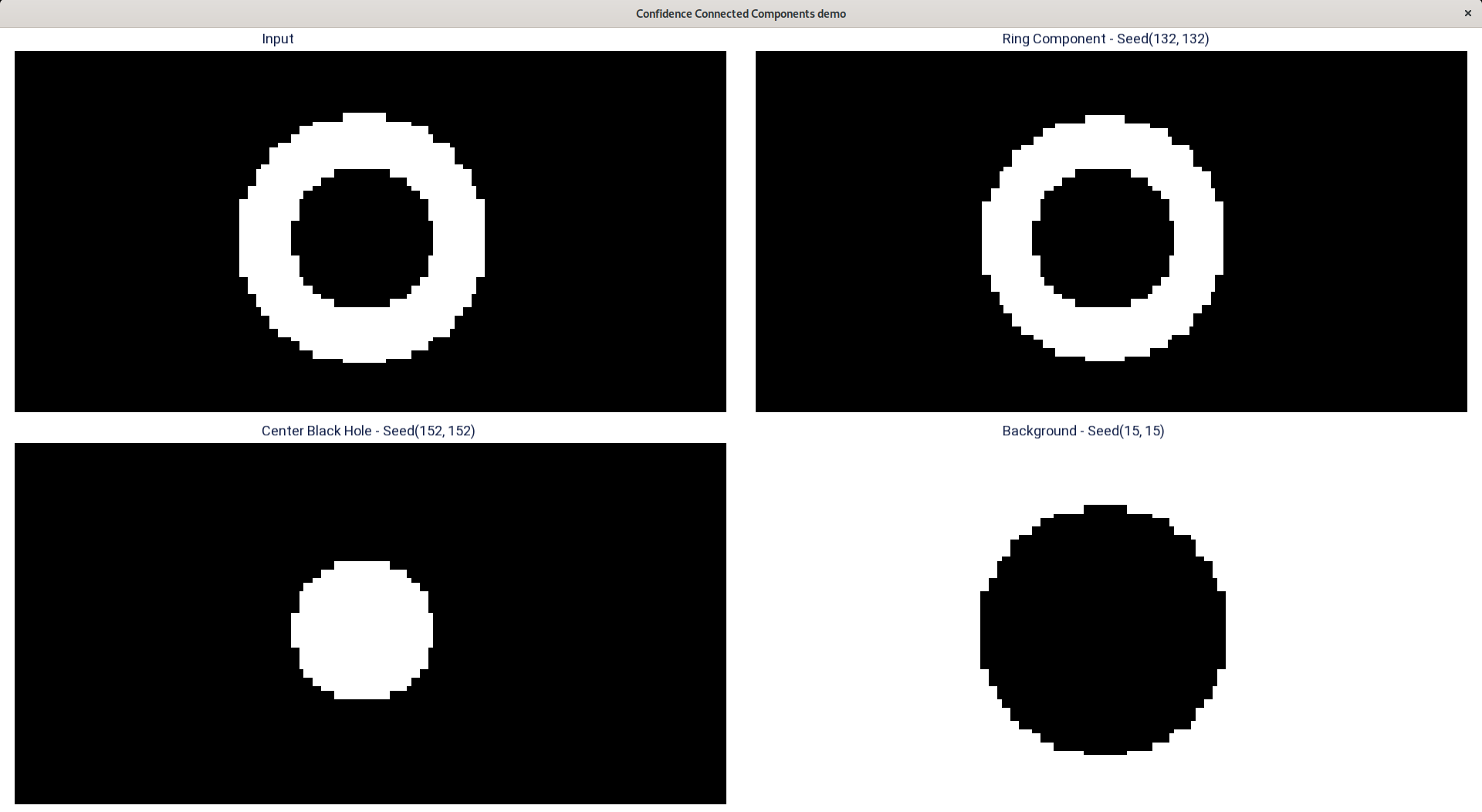A high-performance general-purpose compute library

Segment image based on similar pixel characteristics. More...

## Functions

AFAPI array confidenceCC (const array &in, const array &seeds, const unsigned radius, const unsigned multiplier, const int iter, const double segmentedValue)
C++ Interface for confidence connected components.

AFAPI array confidenceCC (const array &in, const array &seedx, const array &seedy, const unsigned radius, const unsigned multiplier, const int iter, const double segmentedValue)
C++ Interface for confidence connected components.

AFAPI array confidenceCC (const array &in, const size_t num_seeds, const unsigned *seedx, const unsigned *seedy, const unsigned radius, const unsigned multiplier, const int iter, const double segmentedValue)
C++ Interface for confidence connected components.

AFAPI af_err af_confidence_cc (af_array *out, const af_array in, const af_array seedx, const af_array seedy, const unsigned radius, const unsigned multiplier, const int iter, const double segmented_value)
C Interface for confidence connected components.

## Detailed Description

Segment image based on similar pixel characteristics.

This filter is similar to af::regions() (connected components) with additional criteria for segmentation. In af::regions(), all connected (af_connectivity) pixels connected are considered to be a single component. In this variation of connected components, pixels having similar pixel statistics of the neighborhoods around a given set of seed points are grouped together.

The parameter radius determines the size of neighborhood around a seed point.

Mean ( $$\mu$$) and Variance ( $$\sigma^2$$) are the pixel statistics that are computed across all neighborhoods around the given set of seed points. The pixels which are connected to seed points and lie in the confidence interval ( $$[\mu - \alpha * \sigma, \mu + \alpha * \sigma]$$ where $$\alpha$$ is the parameter multiplier) are grouped. multiplier can be used to control the width of the confidence interval.

This filter follows an iterative approach for fine tuning the segmentation. An initial segmenetation followed by a finite number (iter) of segmentations are performed. The user provided parameter iter is only a request and the algorithm can prempt the execution if $$\sigma^2$$ approaches zero. The initial segmentation uses the mean and variance calculated from the neighborhoods of all the seed points. For subsequent segmentations, all pixels in the previous segmentation are used to re-calculate the mean and variance (as opposed to using the pixels in the neighborhood of the seed point).

Given below is a sample output for segmenting three different regions of a donut using single seed.## ◆ af_confidence_cc()

 AFAPI af_err af_confidence_cc ( af_array * out, const af_array in, const af_array seedx, const af_array seedy, const unsigned radius, const unsigned multiplier, const int iter, const double segmented_value )

C Interface for confidence connected components.

Parameters
 [out] out is the output af_array having the connected components [in] in is the input image, expects non-integral (float/double) typed af_array [in] seedx is an af_array of x coordinates of the seed points [in] seedy is an af_array of y coordinates of the seed points [in] radius is the neighborhood region to be considered around each seed point [in] multiplier controls the threshold range computed from the mean and variance of seed point neighborhoods [in] iter is number of iterations [in] segmented_value is the value to which output array valid pixels are set to.
Returns
AF_SUCCESS if the execution is successful, otherwise an appropriate error code is returned.

## ◆ confidenceCC() [1/3]

 AFAPI array confidenceCC ( const array & in, const array & seeds, const unsigned radius, const unsigned multiplier, const int iter, const double segmentedValue )

C++ Interface for confidence connected components.

Parameters
 [in] in is the input image, expects non-integral (float/double) typed af_array [in] seeds is an af::array of x & y coordinates of the seed points with coordinate values along columns of this af::array i.e. they are not stored in interleaved fashion. [in] radius is the neighborhood region to be considered around each seed point [in] multiplier controls the threshold range computed from the mean and variance of seed point neighborhoods [in] iter is number of iterations [in] segmentedValue is the value to which output array valid pixels are set to.
Returns
out is the output af_array having the connected components

## ◆ confidenceCC() [2/3]

 AFAPI array confidenceCC ( const array & in, const array & seedx, const array & seedy, const unsigned radius, const unsigned multiplier, const int iter, const double segmentedValue )

C++ Interface for confidence connected components.

Parameters
 [in] in is the input image, expects non-integral (float/double) typed af_array [in] seedx is an af::array of x coordinates of the seed points [in] seedy is an af::array of y coordinates of the seed points [in] radius is the neighborhood region to be considered around each seed point [in] multiplier controls the threshold range computed from the mean and variance of seed point neighborhoods [in] iter is number of iterations [in] segmentedValue is the value to which output array valid pixels are set to.
Returns
out is the output af_array having the connected components

## ◆ confidenceCC() [3/3]

 AFAPI array confidenceCC ( const array & in, const size_t num_seeds, const unsigned * seedx, const unsigned * seedy, const unsigned radius, const unsigned multiplier, const int iter, const double segmentedValue )

C++ Interface for confidence connected components.

Parameters
 [in] in is the input image, expects non-integral (float/double) typed af_array [in] num_seeds is the total number of seeds [in] seedx is an array of x coordinates of the seed points [in] seedy is an array of y coordinates of the seed points [in] radius is the neighborhood region to be considered around each seed point [in] multiplier controls the threshold range computed from the mean and variance of seed point neighborhoods [in] iter is number of iterations [in] segmentedValue is the value to which output array valid pixels are set to.
Returns
out is the output af_array having the connected components